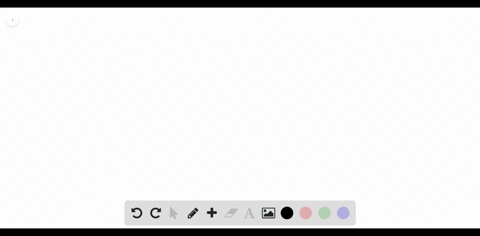### How many hydrogen atoms are in each of the follow…

01:32Manhattan College
Problem 50

Determine the molecular and empirical formulas of the following: (a) the organic solvent benzene, which has six carbon atoms and six hydrogen atoms; (b) the compound silicon tetrachloride, which has a silicon atom and four chlorine atoms and is used in the manufacture of computer chips; (c) the reactive substance diborane, which has two boron atoms and six hydrogen atoms; (d) the sugar called glucose, which has six carbon atoms, twelve hydrogen atoms, and six oxygen atoms.

See step for solution

## Discussion

You must be signed in to discuss.

## Video Transcript

All right, guys. We're doomed from 50 attractor too. And chemistry Central plans. So determine the molecular empirical formers of the following day. The organic solvent benzene, which has six carbon atoms and 600 names. So, eh, to be a 67 Malaysian form, we're goingto have C six h six because we have six carbon atoms, six hydrogen. And for the elect for the empirical formula, we have a 1 to 1 ratio between carbon and hydrogen. So we can write that a c h. So be it the compound silicon tetrachloride, which has a silicon atom for chlorine atoms. So silicon tetrachloride Oh, be a one silicon. And for Koreans, we can't reduce us anymore. So the empirical formula is the same as thie molecular formula. So see the reactive substance that boring, which has two born arms and 600 so be to a sticks is molecular. So we done so so six divided by two. That's going to be three. So they're in a 123 a ratio. So a pair of corn is going to be aged three the the sugar called glucose. So this has six carbon 12. How Gen six oxygen we can reduce that. So are carbon isn't a 1 to 2 toe one ratio with carbon, hydrogen and auction respectively. So that's so that's gonna be our empirical formula. So the G So molecular farmers always have the molecule physically looks. Thean parent form is more of a ratio between in specific items.